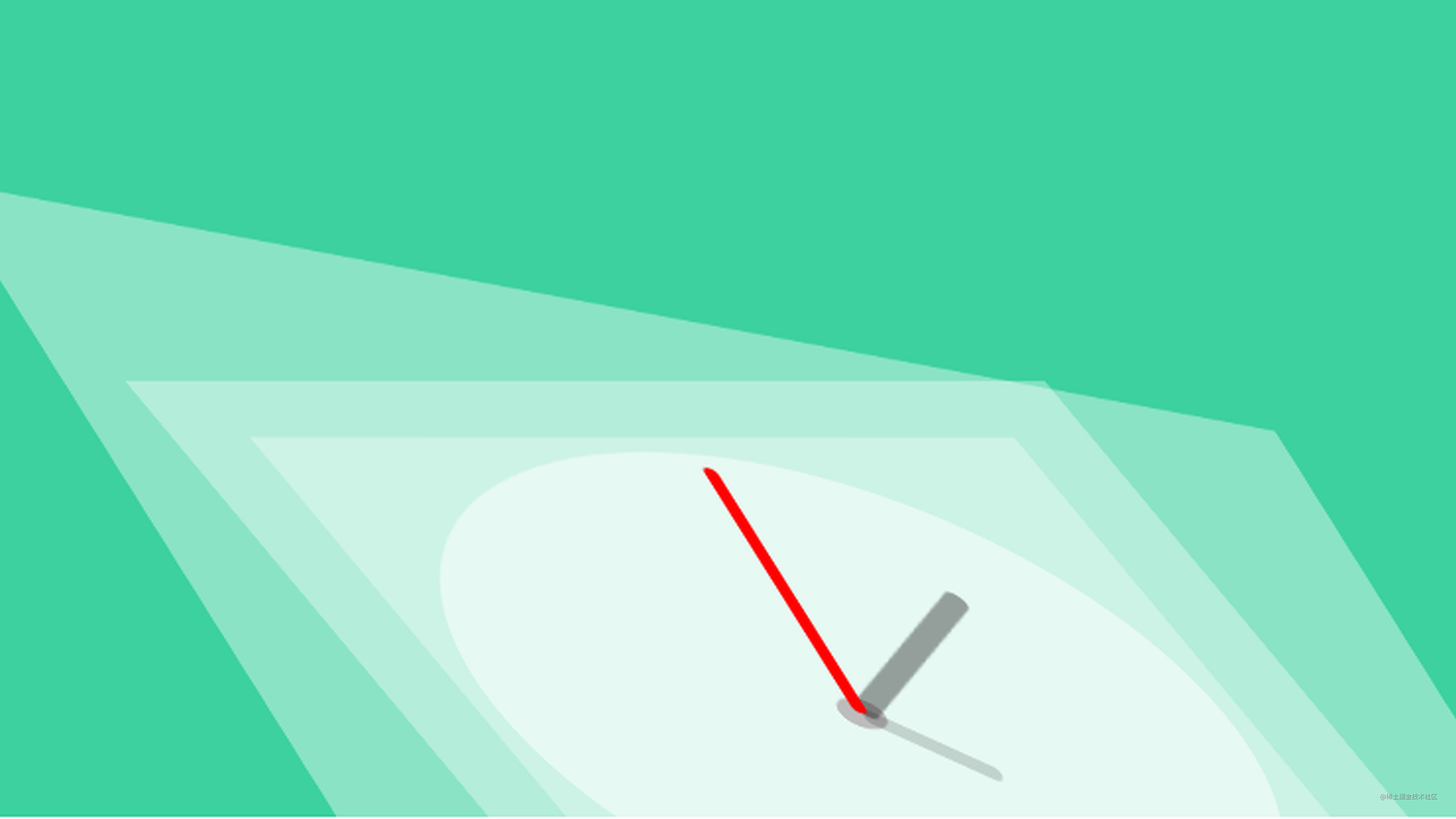# 时钟——CSS 动画# 时钟

## HTML

``````<article class="clock">
<div class="hours-container">
<div class="hours"></div>
</div>
<div class="minutes-container">
<div class="minutes"></div>
</div>
<div class="seconds-container">
<div class="seconds"></div>
</div>
</article>

## 钟面

``````.clock {
background: #fff url(/images/posts/clocks/ios_clock.svg) no-repeat center;
background-size: 88%;
height: 20em;
position: relative;
width: 20em;
}

.clock.simple:after {
background: #000;
content: "";
position: absolute;
left: 50%;
top: 50%;
transform: translate(-50%, -50%);
width: 5%;
height: 5%;
z-index: 10;
}

``````.minutes-container, .hours-container, .seconds-container {
position: absolute;
top: 0;
right: 0;
bottom: 0;
left: 0;
}

## 时针

``````.hours {
background: #000;
height: 20%;
left: 48.75%;
position: absolute;
top: 30%;
transform-origin: 50% 100%;
width: 2.5%;
}

## 分针

``````.minutes {
background: #000;
height: 40%;
left: 49%;
position: absolute;
top: 10%;
transform-origin: 50% 100%;
width: 2%;
}

## 秒针

``````.seconds {
background: #000;
height: 45%;
left: 49.5%;
position: absolute;
top: 14%;
transform-origin: 50% 80%;
width: 1%;
z-index: 8;
}

## 动画

``````@keyframes rotate {
100% {
transform: rotateZ(360deg);
}
}

``````.hours-container {
animation: rotate 43200s infinite linear;
}
.minutes-container {
animation: rotate 3600s infinite linear;
}
.seconds-container {
animation: rotate 60s infinite linear;
}

## 添加停顿

``````.minutes-container {
animation: rotate 3600s infinite steps(60);
}
.seconds-container {
animation: rotate 60s infinite steps(60);
}

## 正确的时间

``````/*
* Starts any clocks using the user's local time
* From: cssanimation.rocks/clocks
*/
function initLocalClocks() {
// Get the local time using JS
var date = new Date;
var seconds = date.getSeconds();
var minutes = date.getMinutes();
var hours = date.getHours();

// Create an object with each hand and it's angle in degrees
var hands = [
{
hand: 'hours',
angle: (hours * 30) + (minutes / 2)
},
{
hand: 'minutes',
angle: (minutes * 6)
},
{
hand: 'seconds',
angle: (seconds * 6)
}
];
// Loop through each of these hands to set their angle
for (var j = 0; j < hands.length; j++) {
var elements = document.querySelectorAll('.' + hands[j].hand);
for (var k = 0; k < elements.length; k++) {
elements[k].style.webkitTransform = 'rotateZ('+ hands[j].angle +'deg)';
elements[k].style.transform = 'rotateZ('+ hands[j].angle +'deg)';
// If this is a minute hand, note the seconds position (to calculate minute position later)
if (hands[j].hand === 'minutes') {
elements[k].parentNode.setAttribute('data-second-angle', hands[j + 1].angle);
}
}
}
}

## 对齐秒针和分针

``````if (hands[j].hand === 'minutes') {
elements[k].parentNode.setAttribute('data-second-angle', hands[j + 1].angle);
}

``````/*
* Set a timeout for the first minute hand movement (less than 1 minute), then rotate it every minute after that
*/
function setUpMinuteHands() {
// Find out how far into the minute we are
var containers = document.querySelectorAll('.minutes-container');
var secondAngle = containers.getAttribute("data-second-angle");
if (secondAngle > 0) {
// Set a timeout until the end of the current minute, to move the hand
var delay = (((360 - secondAngle) / 6) + 0.1) * 1000;
setTimeout(function() {
moveMinuteHands(containers);
}, delay);
}
}

/*
* Do the first minute's rotation
*/
function moveMinuteHands(containers) {
for (var i = 0; i < containers.length; i++) {
containers[i].style.webkitTransform = 'rotateZ(6deg)';
containers[i].style.transform = 'rotateZ(6deg)';
}
// Then continue with a 60 second interval
setInterval(function() {
for (var i = 0; i < containers.length; i++) {
if (containers[i].angle === undefined) {
containers[i].angle = 12;
} else {
containers[i].angle += 6;
}
containers[i].style.webkitTransform = 'rotateZ('+ containers[i].angle +'deg)';
containers[i].style.transform = 'rotateZ('+ containers[i].angle +'deg)';
}
}, 60000);
}

## 添加弹跳

``````/*
* Move the second containers
*/
function moveSecondHands() {
var containers = document.querySelectorAll('.seconds-container');
setInterval(function() {
for (var i = 0; i < containers.length; i++) {
if (containers[i].angle === undefined) {
containers[i].angle = 6;
} else {
containers[i].angle += 6;
}
containers[i].style.webkitTransform = 'rotateZ('+ containers[i].angle +'deg)';
containers[i].style.transform = 'rotateZ('+ containers[i].angle +'deg)';
}
}, 1000);
}

``````.minutes-container {
transition: transform 0.3s cubic-bezier(.4,2.08,.55,.44);
}
.seconds-container {
transition: transform 0.2s cubic-bezier(.4,2.08,.55,.44);
}

（完）